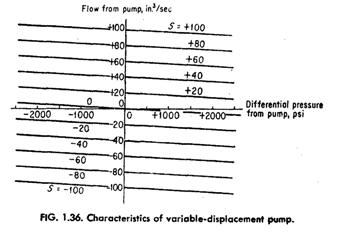﻿ Variable Displacement Pump Flow and Pressure Characteristics – Hydraulic Schematic Troubleshooting

## Variable Displacement Pump Flow and Pressure Characteristics

Generally, the flow and pressure characteristics of variable-displacement pumps are given as constants assuming linear characteristics. As stated before, for high-pressure operation with low-viscosity fluids, the pump characteristics may become more nonlinear. In this case, the nonlinearities should be considered in the system servo analysis work. Complete pump characteristics of flow and pressure can also be given in the same form as for control valves. Figure 1.36 gives the pump characteristics of flow and pressure, considering that the pump is supplying power both to a load and for an overhauling load, at which time the pump is absorbing power in retarding the load.

As with control valves, the characteristics of a pump can be represented by gradients which may be obtained from Fig. 1.36. The flow gradient is the slope of the flow versus stroke curve. by considering the pressure const.ant. The pressure gradient is the slope of the pressure versus stroke curve for constant flow. The leakage coefficient Ls can be obtained by dividing the flow gradient by the pressure gradient or by the slope of the constant stroke curves of Fig. 1.36.